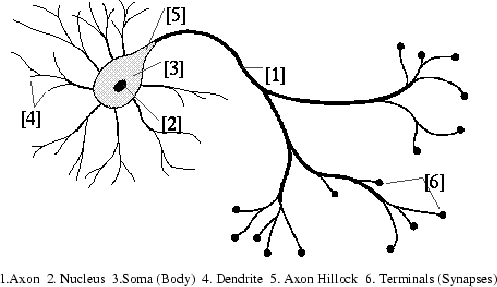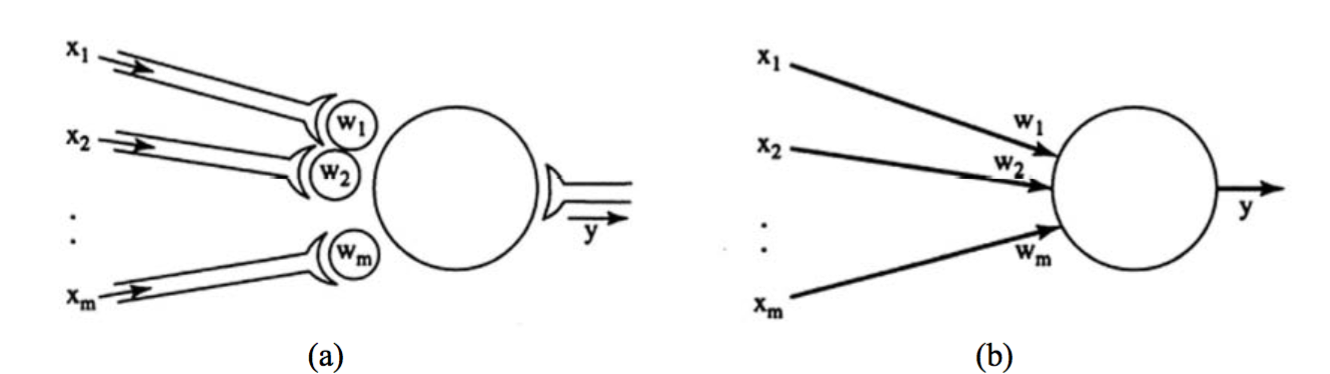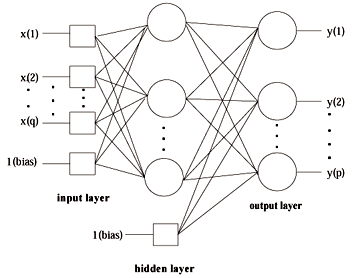Today’s topic is how to implement an artificial neural network.

Well, what is an artificial neural network? First, let’s see what is a natural neural network. Look at this guyfigure (a). This is the source of humans’ intelligence which is called a natural neural network. We humans want to make machines mimic our intelligence, so we figure out an artificial neural network to mimic the natural neural network. The artificial neural network is so popular that we often drop the “artificial” and simply call it the neural network. You will find soon that neural networks try to mimic everything natural neural networks do, from construction to training.Let’s say now we are going to implement a neural network which can solve arithmetical problems.

## How to construct a neural network

What shall we do first? First, we need to construct a neural network. Here we go! Look at the natural neural network, it has neurons, right? We copy it!These nodes in the neural networkfigure (b) are called artificial neurons, simply neurons. The edges denote connections between neurons. And the weights on edges represent the biological synaptic strengths in a natural neuron. Each input $$x_i$$ is multiplied by its corresponding weight $$w_i$$, then the product $$x_i⋅w_i$$ is fed into the body of the neuron. The neuron adds up all the products for $$i = 1, …, m$$. It looks like this:
$$\mathbf{x} = (x_1, x_2, \cdots, x_m)$$
$$\mathbf{w} = (w_1, w_2, \cdots, w_m)$$
$$\mathbf{x}\mathbf{w} = x_1w_1 + x_2w_2 + \cdots + x_mw_m$$
$$net = \mathbf{x}\mathbf{w}$$

So far so good! If you are familiar with natural neural networks, you may find currently some important pieces are missing.

First, somehow, a natural neuron always performs some complex process for the incoming electrochemical signals. Think about it. If your neurons just simply add up all the incoming signals, you cannot be so smart:-) Right? The question is how to mimic this behavior in our neural network. Any ideas? Note that any process is a mapping from the input to output, in mathematics, we call it what? Yes, function! Here, we define an activation function to simulate this behavior. Very often, we choose some nonlinear functions like the sigmoid function which is shown below:
$$y = f(net)$$
$$f(x) = \frac{1}{1 + e^{-x}}$$

Another problem is in a natural neural network, there is always a threshold for the excitation of the neuron. That is to say, if the incoming signals are so weak, a natural neuron may ignore them. We can do the same thing in our neural network. That’s a simple trick in mathematics. We just need to simply add a constant term in the sum formula. It looks like this:
$$\mathbf{x}\mathbf{w} = x_1w_1 + x_2w_2 + \cdots + x_mwm + x{m+1}w{m+1}, ~~ where ~~ x{m+1}=1$$

$$net = \mathbf{x}\mathbf{w}$$

where the $$w_{m+1}$$ is essentially the threshold.

Well yet, we are almost done! Let’s now construct a 3-layers neural network which is the most common case.

## The power of a neural network

Look at this neural networkfigure (c), we have Ni inputs and No outputs. Thus we can always get something as long as we feed the network some inputs. A very good thing is as long as we have enough neurons in the second layer which is called a hidden layer, the neural network can approximate any function no matter how complex it would be. Very nice property! It means that the neural network can learn anything as long as it’s learnable.## How to train a neural network

Then, let’s go to the next step. How to train a neural network to make it as smart as a natural neural network? Again, neural networks try to mimic everything natural neural networks do. Think about it. How do young children learn arithmetic? They learn from examples! They may need to practice hundreds of arithmetical problems before they are good at arithmetic.

Neural networks do pretty much the same thing. They learn from training data. Every time a neural network makes a try, we supervise it, if it dose well, we encourage it; otherwise, we discourage it and update its weights. It may need to practice all the examples again and again until it never makes mistakes or only makes a few mistakes in these examples. Finally, we would say “as a neural network, you definitely have already learned arithmetic!”

That’s the basic idea that how we implement a neural network. For a specific python implementation of a neural network, please click here.